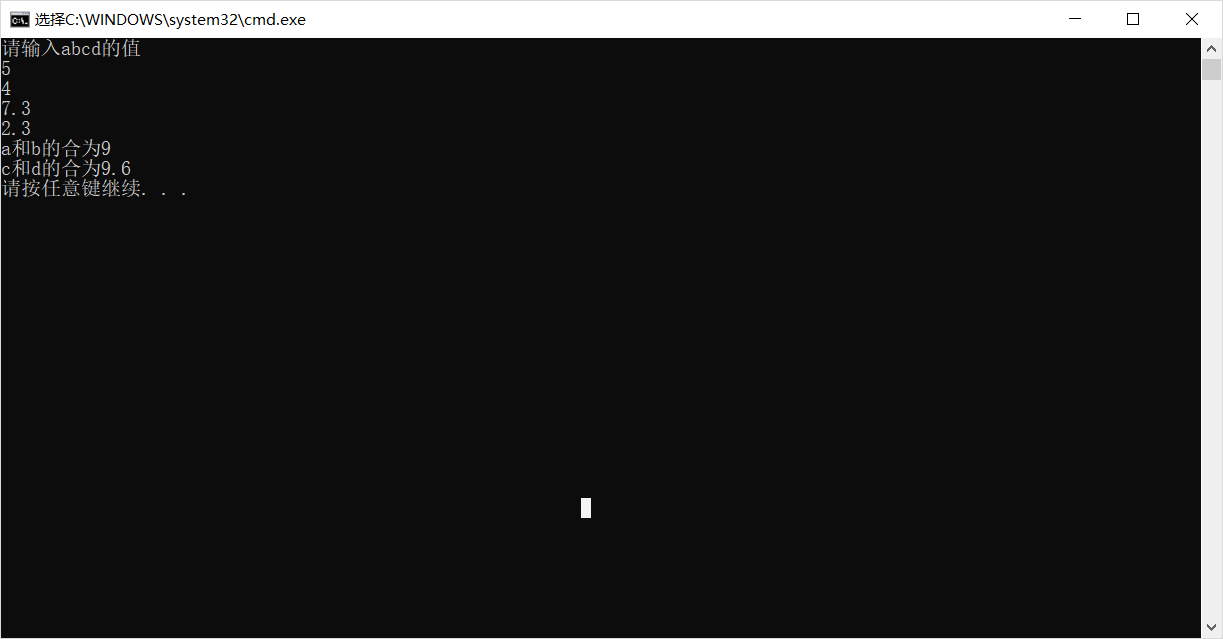# 函数的重载

## 3：函数重载的实现

#include<iostream>
using namespace std;
int abc(int a, int b)
{
int sum;
sum = a + b;
return sum;
}
float abc(float a, float b)
{
float sum;
sum = a + b;
return sum;
}


#include<iostream>
using namespace std;
int abc(int a, int b)
{
int sum;
sum = a + b;
return sum;
}
float abc(float a, float b)
{
float sum;
sum = a + b;
return sum;
}
void main()
{
int a, b;
float c, d;
double sum1,sum2;
cout << "请输入abcd的值" << endl;
cin >> a  >> b  >> c  >> d;
sum1 = abc(a, b);
sum2 = abc(c , d);
cout <<"a和b的合为"<< sum1 << endl<<"c和d的合为" << sum2 << endl;
}## 4使用函数重载可以避免什么样的问题

posted @ 2019-09-15 09:16  不见风雨不见你  阅读(4171)  评论(0编辑  收藏  举报